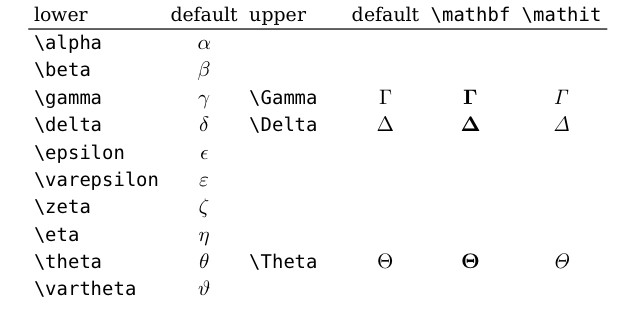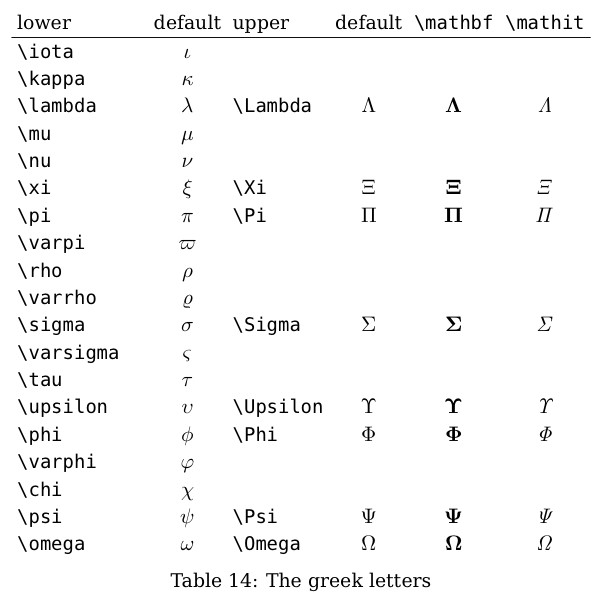# Latex常见用法

Posted by jjx on September 4, 2016

1、上下标的使用

下标的输入命令为：
$_{内容}$



2、插入分数

Latex插入分数的命令是\frac{分子}{分母}。输入如下代码：

$h_{0}(x)=\frac{1}{1+e^{-\theta}}$

$h_{0}(x)=\frac{1}{1+e^{-\theta}}$

其中${x1,x2,x3}$为输入单元，x$_0$称为偏置单元（bias unit），$x_0=1$，{$x_0$，$x_1$，$x_2$，$x_3$}称为连接权重。其中$h_0(x)=\frac{1}{1+e^{-\theta^{t}*x}}$。



3、希腊字母4、矩阵表示

$\left( \begin{array}{ccc} 1 & 2 & 3 \\ 4 & 5 & 6 \\ 7 & 8 & 9 \\ \end{array} \right)$

$\left( \begin{array}{ccc} 1 & 2 & 3 \\ 4 & 5 & 6 \\ 7 & 8 & 9 \\ \end{array} \right)$

\sum_{i=1}^{m}


\lim_{n\to \infty}

$\lim_{n\to \infty}$